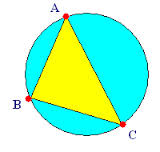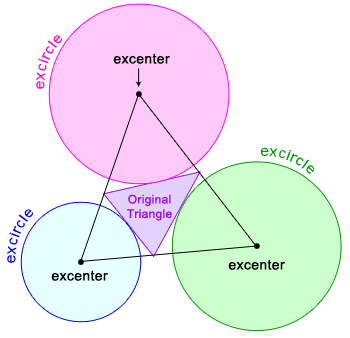×#### Thank you for registering.

One of our academic counsellors will contact you within 1 working day.

Click to Chat

1800-1023-196

+91-120-4616500

CART 0

• 0

MY CART (5)

Use Coupon: CART20 and get 20% off on all online Study Material

ITEM
DETAILS
MRP
DISCOUNT
FINAL PRICE
Total Price: Rs.

There are no items in this cart.
Continue Shopping```Properties and solutions of TrianglesProperties and solutions of Triangles is a vital component in the IIT JEE mathematics syllabus. With the help of practice, a person can get good hold on these topics which easily fetch 2-3 questions in IIT JEE. This head also comprises various formulae and rules like the sin rule, cosine rule, tangent rule etc. Many a times questions based on application of these results are often asked in exams.

Various sub heads which come under this topic are enlisted below:

Solution of Triangles

Circles Connected With Triangle

Solved Examples of Solution of Triangle

These topics have been discussed in detail in the coming sections. Here, we shall just give an outline of these heads.

Solution of Triangles:

In a triangle ABC, the side opposite to angle A is denoted by ‘a’, that opposite to angle B is denoted by ‘b’ and similarly the one opposite to angle C is

denoted by ‘c’.Here, we shall discuss the relationship between the sides and angles of triangle ABC.

Sine Rule:

This rule states that the sides of any triangle are proportional to the sines of the angle opposite to them in ?ABC, i.e.

a/ sin A = b/ sin B = c/ sin C

This value in turn is also equal to 2R, where ‘R’ is the circumradius of the triangle ABC.

The sine rule can also be written as

(sin A)/a = (sin B)/b = (sin C)/c

Cosine Rule:

Consider a triangle ABC with sides opposite to A, B and C being ‘a’, ‘b’ and ‘c’. Then we have,

cos A =  (b2 + c2 –a2) / 2bc

cos B =  (c2 + a2 - b2) / 2ac

cos C =  (a2 + b2 –c2) / 2ab

The cosine rule can be used to find the sides of the triangle.

Projection Formulae:

In any triangle ABC, the following results hold true:

a = b cos C + c cos B

b = c cos A + a cos C

c = a cos B + b cos A

Area of a triangle:

Area of triangle Δ = 1/2 bc sin A

= 1/2 ca sinB

= 1/2 ab sin C

= √s(s - a) (s - b) (s - c)

Besides these, there are various other trigonometric formulae which have been discussed in the later sections. Those desirous of a deep study of the topic are advised to refer the following sections.

Circles Connected with triangle:

Various types of circles associated with triangle include circumcircle, incircle, escribed circle etc. Various trigonometric formulas associated with these concepts have been reviewed in the later sections. Let’s discuss them in brief here:

Circumcircle of a triangle:

A circle passing through the vertices of a triangle is called the circumcircle of a triangle. The radius of the circumcircle is called the circumradius of the triangle and is denoted by R and is given by the formulae

R = a/2 sin A = b/ 2 sin B = c/2 sin CIncircle of a triangle:

A circle inscribed within the triangle in such a way that it touches all the three sides of the triangle is called an in circle. The centre of the incircle is called the incentre of the triangle.

The radius ‘r’ of the inscribed circle of triangle ABC is given by

r = ?/s

or r = (s-a)tan A/2 = (s-b)tan B/2 =(s-c)tan C/2Various other formulae related formulae related to the topic have been discussed in the later sections.

Escribed Circle:

The circle which touches the side BC and two sides AB and AC produced of a triangle ABC is called the escribed circle opposite to angle A. As depicted in the figure given below, the centres of the escribed circles are called the excentres.The various results related to the ex-radius have been mentioned in the following pages.

We now discuss some of the illustrations based on these concepts:

Illustration:

In a triangle ABC, 2ac sin 1/2 (A-B+C) = ?

Solution:

In a triangle ABC, we know that A+B+C = 180°

Hence, A-B+C = 180° – 2B

Hence, 2ac sin [1/2 (A-B+C)] = 2ac sin (90°–B)

= 2ac cos B

= a2+c2-b2   (using the law of cosines)

Illustration:

Which of the following pieces of data does not uniquely determine an acute angled triangle ABC (where R is the radius of the circumcircle)?

1. a, sin A, sin B                                                  2. a, b, c

3. a, sin B, R                                                       4. a, sin A, R

Solution:

We discuss all the four parts one by one.

1. When a, sin A, sin B are given it becomes possible to find

b = a sin B/ sin A,

c = a sin C/ sin A

So, all the three sides are unique.

2. The three sides can uniquely make an acute angled triangle.

3. a, sin B, R is given and so the data is sufficient for computing the remaining sides and triangles.

B = 2R sin B, sin A = a sin B/b

So, sin C can be determined.

4. a, sin A, R is given, data is in sufficient to find the other sides and angles

b/ sin B = c/ sin C = 2R

Hence, it is clear that this could not determine the exact values of a and b.

Illustration:

Comment on the statement whether it is possible to draw any tangent from the point (5/2, 1) to the circumcirle of the triangle with vertices (1, √3), (1, -√3), (3, √3).

Solution:

Since, (1, √3), (1, -√3), (3, √3) form a right angle triangle at (1, √3)

Therefore, the equation of the circumcircle taking (3, √3) and (1, -√3) as end points of the diameter

So, (x-3) (x-1) + (y - √3) (y + √3) = 0.

This means, x2 - 4x +3 + y2 – 3 = 0.

Hence, x2 - 4x + y2 = 0.

At point (5/2, 1), S1 = 25/4 + 1 -10 < 0

Hence, point (5/2, 1) lies inside the circle.

Since, the point lies inside the circle, so it is not possible to draw any tangent.

You might like to refer some of the related resources listed below:

Look into the Revision Notes on Trigonometry for a quick revision.

Various Recommended Books of Mathematics are just a click away.

To read more, Buy study materials of Trigonometry comprising study notes, revision notes, video lectures, previous year solved questions etc. Also browse for more study materials on Mathematics here.
```### Course Features

• 731 Video Lectures
• Revision Notes
• Previous Year Papers
• Mind Map
• Study Planner
• NCERT Solutions
• Discussion Forum
• Test paper with Video Solution